Related Articles

# Python – Categorical Encoding using Sunbird

• Last Updated : 26 Nov, 2020

The Sunbird library is the best option for feature engineering purposes. In this library, you will get various techniques to handle missing values, outliers, categorical encoding, normalization and standardization, feature selection techniques, etc. It can be installed using the below command:

`pip install sunbird`

## Categorical Encoding

Categorical data is a common type of non-numerical data that contains label values and not numbers. Some examples include:

Colors: White, Black, Green. Cities: Mumbai, Pune, Delhi. Gender: Male, Female.

In order to various encoding techniques we are going to use the below dataset:

## Python3

 `# importing libraries``import` `pandas as pd`` ` `# creating dataset``data ``=` `{``'Subject'``: [``'s1'``, ``'s2'``, ``'s3'``, ``'s1'``, ``'s4'``,``                        ``'s3'``, ``'s2'``, ``'s1'``, ``'s2'``, ``'s4'``, ``'s1'``],``        ``'Target'``: [``1``, ``0``, ``1``, ``1``, ``1``, ``0``, ``0``, ``1``, ``1``, ``1``, ``0``]}`` ` `# convert to dataframe``df ``=` `pd.DataFrame(data)`` ` `# display the dataset``df`

Output: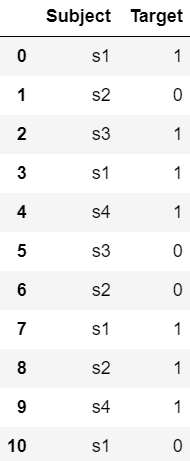#### Various encoding algorithms available in Categorical Encoding are:

1) Frequency Encoding:

Frequency Encoding uses the frequency of the categories in data. In this method, we encode the categories with their frequency.

If we take the example of a Country in that frequency of India is 40 then we encode it with 40.

The disadvantage of this method is supposed two categories have the same number of frequencies then the encoded value for both the categories is the same.

Syntax:

```from sunbird.categorical_encoding import frequency_encoding
frequency_encoding(dataframe, 'categorical-column')```

Example:

## Python3

 `# importing libraries``from` `sunbird.categorical_encoding ``import` `frequency_encoding``import` `pandas as pd`` ` `# creating dataset``data ``=` `{``'Subject'``: [``'s1'``, ``'s2'``, ``'s3'``, ``'s1'``, ``'s4'``,``                    ``'s3'``, ``'s2'``, ``'s1'``, ``'s2'``, ``'s4'``, ``'s1'``],``        ``'Target'``: [``1``, ``0``, ``1``, ``1``, ``1``, ``0``, ``0``, ``1``, ``1``, ``1``, ``0``]}`` ` `df ``=` `pd.DataFrame(data)`` ` `# applying frequency encoding``frequency_encoding(df, ``'Subject'``)`` ` `# display the dataset``df`

Output: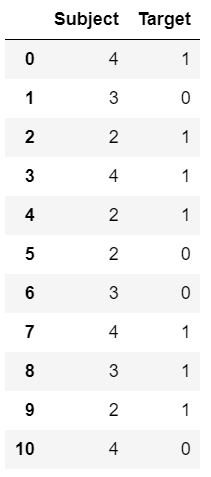2) Target Guided Encoding:

In this encoding, Features are replaced with a blend of the posterior probability of the target given a particular categorical value and the prior probability of the target over all the training data. This method orders the labels according to their target.

Syntax:

```from sunbird.categorical_encoding import target_guided_encoding
target_guided_encoding(dataframe, 'categorical-column', 'target-column')```

Example:

## Python3

 `# importing libraries``from` `sunbird.categorical_encoding ``import` `target_guided_encoding``import` `pandas as pd`` ` `# creating dataset``data ``=` `{``'Subject'``: [``'s1'``, ``'s2'``, ``'s3'``, ``'s1'``, ``'s4'``,``                    ``'s3'``, ``'s2'``, ``'s1'``, ``'s2'``, ``'s4'``, ``'s1'``],``        ``'Target'``: [``1``, ``0``, ``1``, ``1``, ``1``, ``0``, ``0``, ``1``, ``1``, ``1``, ``0``]}`` ` `df ``=` `pd.DataFrame(data)`` ` `# applying target guided encoding``target_guided_encoding(df, ``'Subject'``, ``'Target'``)`` ` `# display the dataset``df`

Output: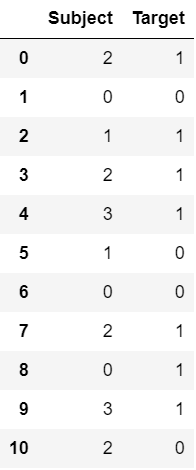3) Probability Ratio Encoding:

Probability Ratio Encoding is based on the predictive power of an independent variable in relation to the dependent variable with respect to the ratio of good and bad probability is used.

Syntax:

```from sunbird.categorical_encoding import probability_ratio_encoding
probability_ratio_encoding(dataframe, 'categorical-column', 'target-column')```

Example:

## Python3

 `# importing libraries``from` `sunbird.categorical_encoding ``import` `probability_ratio_encoding``import` `pandas as pd`` ` `# creating dataset``data ``=` `{``'Subject'``: [``'s1'``, ``'s2'``, ``'s3'``, ``'s1'``, ``'s4'``,``                    ``'s3'``, ``'s2'``, ``'s1'``, ``'s2'``, ``'s4'``, ``'s1'``],``        ``'Target'``: [``1``, ``0``, ``1``, ``1``, ``1``, ``0``, ``0``, ``1``, ``1``, ``1``, ``0``]}`` ` `df ``=` `pd.DataFrame(data)`` ` `# applying probability ratio encoding``probability_ratio_encoding(df, ``'Subject'``, ``'Target'``)`` ` `# display the dataset``df`

Output: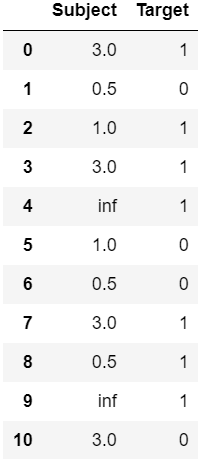4) Mean Encoding:

This type of encoding captures information within the label, therefore rendering more predictive features, it creates a monotonic relationship between the variable and the target. However, it may cause over-fitting in the model.

Syntax:

```from sunbird.categorical_encoding import mean_encoding
mean_encoding(dataframe, 'categorical-column', 'target-column')```

Example:

## Python3

 `# importing libraries``from` `sunbird.categorical_encoding ``import` `mean_encoding``import` `pandas as pd`` ` `# creating dataset``data ``=` `{``'Subject'``: [``'s1'``, ``'s2'``, ``'s3'``, ``'s1'``, ``'s4'``, ``'s3'``,``                    ``'s2'``, ``'s1'``, ``'s2'``, ``'s4'``, ``'s1'``],``        ``'Target'``: [``1``, ``0``, ``1``, ``1``, ``1``, ``0``, ``0``, ``1``, ``1``, ``1``, ``0``]}`` ` `df ``=` `pd.DataFrame(data)`` ` `# applying mean encoding``mean_encoding(df, ``'Subject'``, ``'Target'``)`` ` `# display the dataset``df`

Output: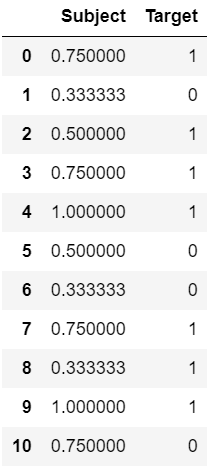5) One Hot Encoding:

In this encoding method, we encode values to 0 or 1 depending on the presence or absence of that category. The number of features or dummy variables depending on the number of categories present in the encoded feature.

For example, the temperature of the water can have three categories warm, hot, cold so the number of dummy variables or features generated will be 3.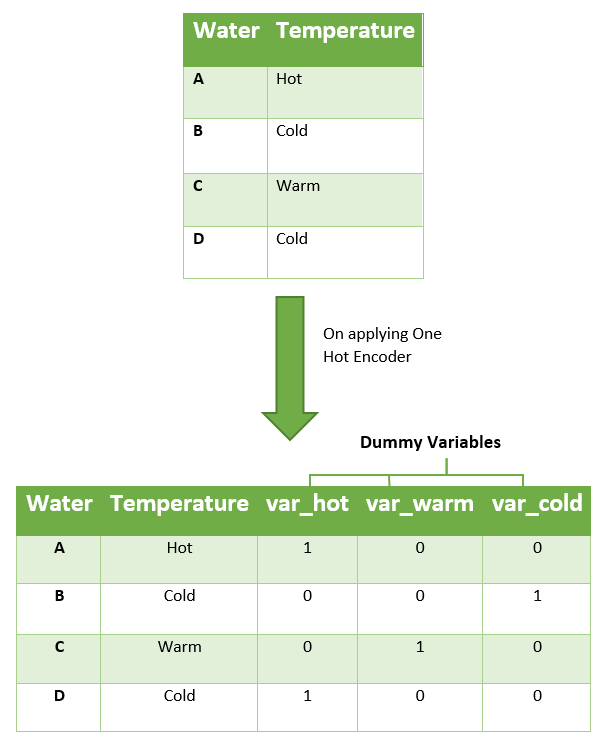Syntax:

```from sunbird.categorical_encoding import one_hot
one_hot(dataframe, 'categorical-column')```

Example 1:

## Python3

 `# importing libraries``import` `pandas as pd``from` `sunbird.categorical_encoding ``import` `one_hot`` ` `# creating dataset``data ``=` `{``'Water'``: [``'A'``, ``'B'``, ``'C'``, ``'D'``, ``'E'``, ``'F'``, ``'G'``],``        ``'Temperature'``: [``'Hot'``, ``'Cold'``, ``'Warm'``, ``'Cold'``,``                        ``'Hot'``, ``'Hot'``, ``'Warm'``]}`` ` `df ``=` `pd.DataFrame(data)`` ` `# applying one hot encoding``one_hot(df, ``'Temperature'``)`` ` `# display the dataset``df`

Output: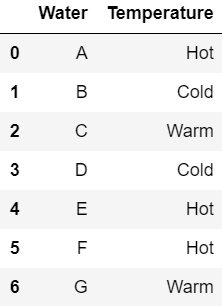Example 2:

## Python3

 `# importing libraries``import` `pandas as pd``from` `sunbird.categorical_encoding ``import` `one_hot`` ` `# creating dataset``data ``=` `{``'Subject'``: [``'s1'``, ``'s2'``, ``'s3'``, ``'s1'``, ``'s4'``, ``'s3'``,``                    ``'s2'``, ``'s1'``, ``'s2'``, ``'s4'``, ``'s1'``],``        ``'Target'``: [``1``, ``0``, ``1``, ``1``, ``1``, ``0``, ``0``, ``1``, ``1``, ``1``, ``0``]}`` ` `df ``=` `pd.DataFrame(data)`` ` `# applying one hot encoding``one_hot(df, ``'Subject'``)`` ` `# display the dataset``df`

Output: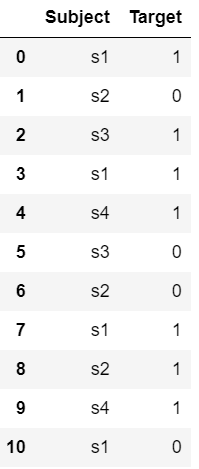6) One Hot Encoding With Multiple Categories:

When we have more categories in a particular categorical feature, after applying one-hot encoding on that feature the number of columns generated by that is also more. In that case, we use one-hot encoding with multi-categories in this encoding method we take more frequent categories.

Here k defines the number of frequent features you want to take. The default value of k is 10.

Syntax:

```from sunbird.categorical_encoding import kdd_cup
kdd_cup(dataframe, 'categorical-column', k=10)```

Example 1:

## Python3

 `# importing libraries``import` `pandas as pd``from` `sunbird.categorical_encoding ``import` `kdd_cup`` ` `# creating dataset``data ``=` `{``'Water'``: [``'A'``, ``'B'``, ``'C'``, ``'D'``, ``'E'``, ``'F'``, ``'G'``],``        ``'Temperature'``: [``'Hot'``, ``'Cold'``, ``'Warm'``, ``'Cold'``,``                        ``'Hot'``, ``'Hot'``, ``'Warm'``]}`` ` `df ``=` `pd.DataFrame(data)`` ` `# applying one hot encoding``kdd_cup(df, ``'Temperature'``, k``=``10``)`` ` `# display the dataset``df`

Output: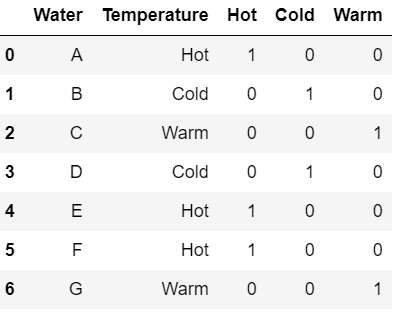Example 2:

## Python3

 `# importing libraries``import` `pandas as pd``from` `sunbird.categorical_encoding ``import` `kdd_cup`` ` `# creating dataset``data ``=` `{``'Subject'``: [``'s1'``, ``'s2'``, ``'s3'``, ``'s1'``, ``'s4'``, ``'s3'``,``                    ``'s2'``, ``'s1'``, ``'s2'``, ``'s4'``, ``'s1'``],``        ``'Target'``: [``1``, ``0``, ``1``, ``1``, ``1``, ``0``, ``0``, ``1``, ``1``, ``1``, ``0``]}`` ` `df ``=` `pd.DataFrame(data)`` ` `# applying one hot encoding``kdd_cup(df, ``'Subject'``, k``=``10``)`` ` `# display the dataset``df`

Output: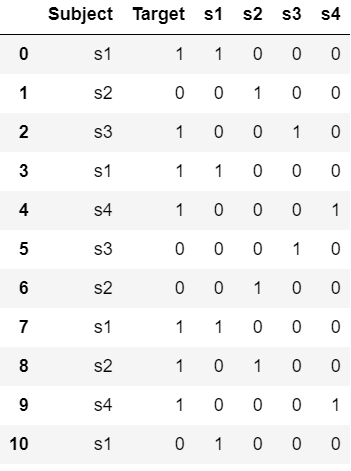Attention reader! Don’t stop learning now. Get hold of all the important Machine Learning Concepts with the Machine Learning Foundation Course at a student-friendly price and become industry ready.

My Personal Notes arrow_drop_up